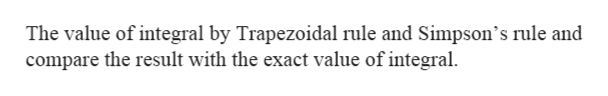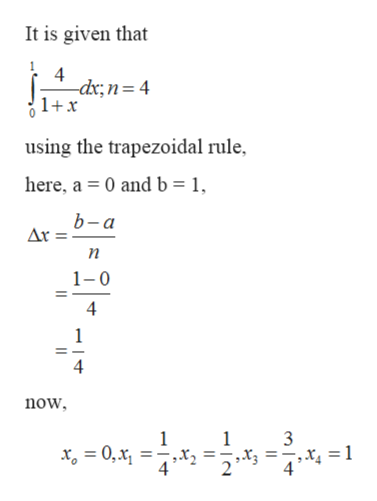# Use the trapezoidal rule and Simpson's rule to approximate the value of the definite integral. Compare your result with the exact value of the integral. (Give your answers correct to 4 decimal places.) 0∫14/(1+x) dx; n=4

Question
4 views

Use the trapezoidal rule and Simpson's rule to approximate the value of the definite integral. Compare your result with the exact value of the integral. (Give your answers correct to 4 decimal places.)

014/(1+x) dx; n=4

check_circle

Step 1

Given: -

Step 2

To find: -help_outlineImage TranscriptioncloseThe value of integral by Trapezoidal rule and Simpson's rule and compare the result with the exact value of integral. fullscreen
Step 3

Calculate:...help_outlineImage TranscriptioncloseIt is given that 4 -dx;n= 4 1+x using the trapezoidal rule, here, a 0 and b = 1, b-a Ar n 1-0 4 1 4 now 1 1 3 X4 = 1 4 4 fullscreen

### Want to see the full answer?

See Solution

#### Want to see this answer and more?

Solutions are written by subject experts who are available 24/7. Questions are typically answered within 1 hour.*

See Solution
*Response times may vary by subject and question.
Tagged in

### Integration